# Selina Solutions Concise Mathematics Class 6 Chapter 33 Data Handling Exercise 33(A)

Selina Solutions Concise Mathematics Class 6 Chapter 33 Data Handling Exercise 33(A) has answers designed by experts to nurture the minds of students about the concepts covered in this chapter. Data Handling is one of the important topics, which would be continued in higher classes. The method of handling the given set of data is the main concept talked about under this exercise. Those who aspire to score good marks, are advised to solve the exercise wise problems, using these solutions. For in-depth knowledge of concepts, Selina Solutions Concise Mathematics Class 6 Chapter 33 Exercise 33(A) free PDF, from the links which are mentioned below.

## Selina Solutions Concise Mathematics Class 6 Chapter 33 Data Handling Exercise 33(A) Download PDF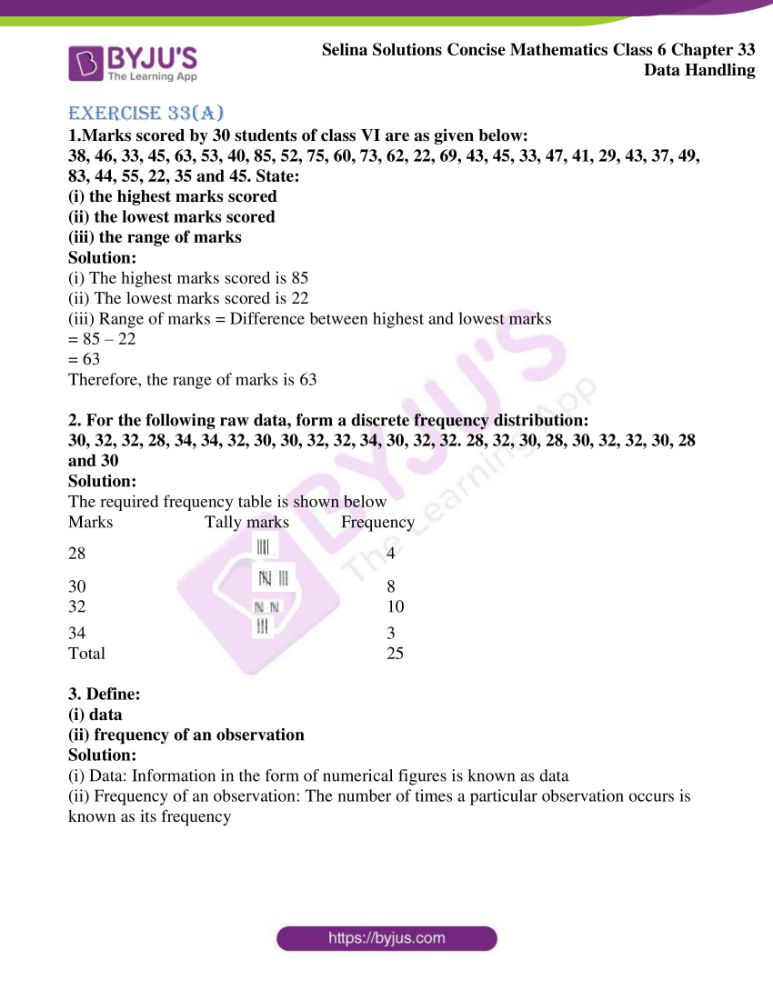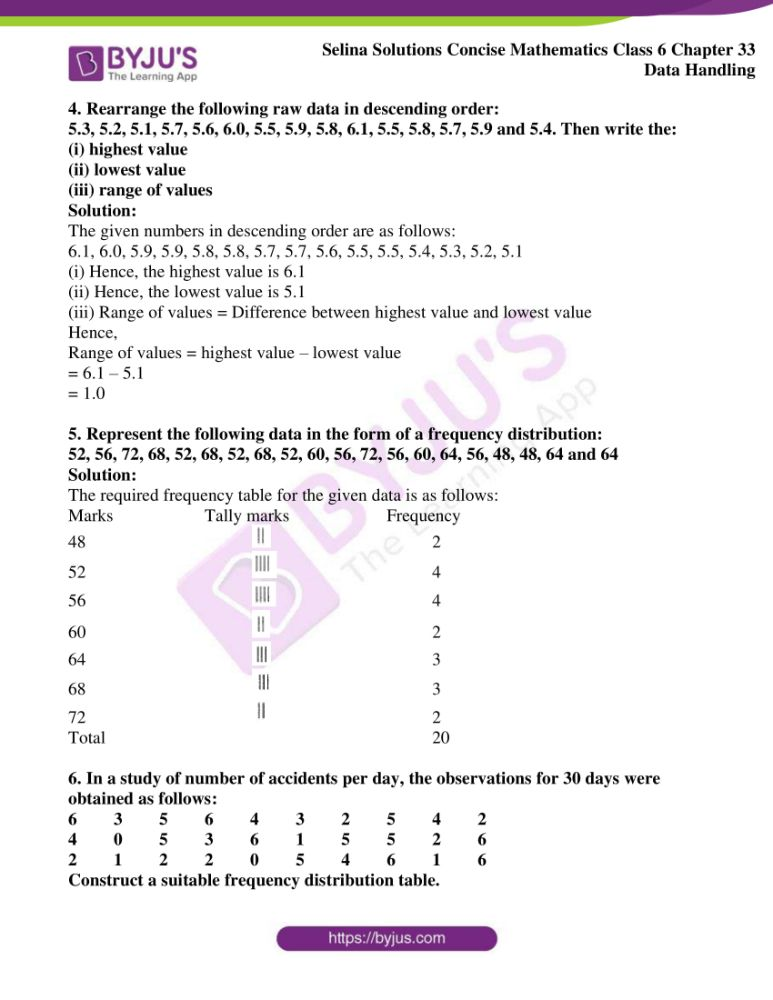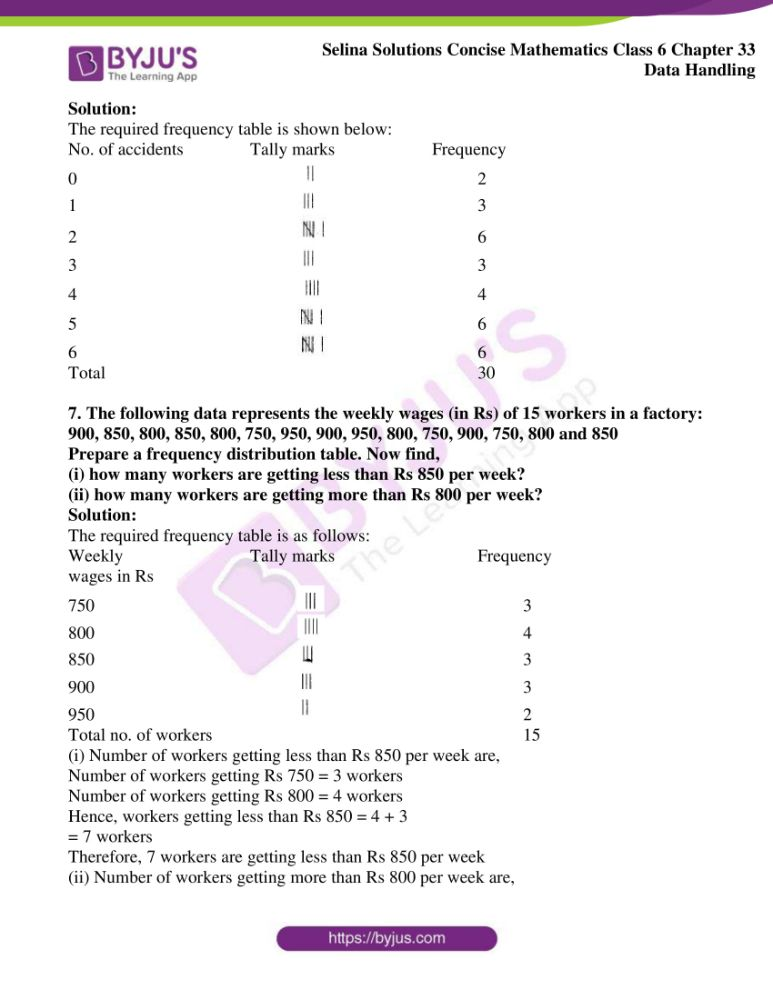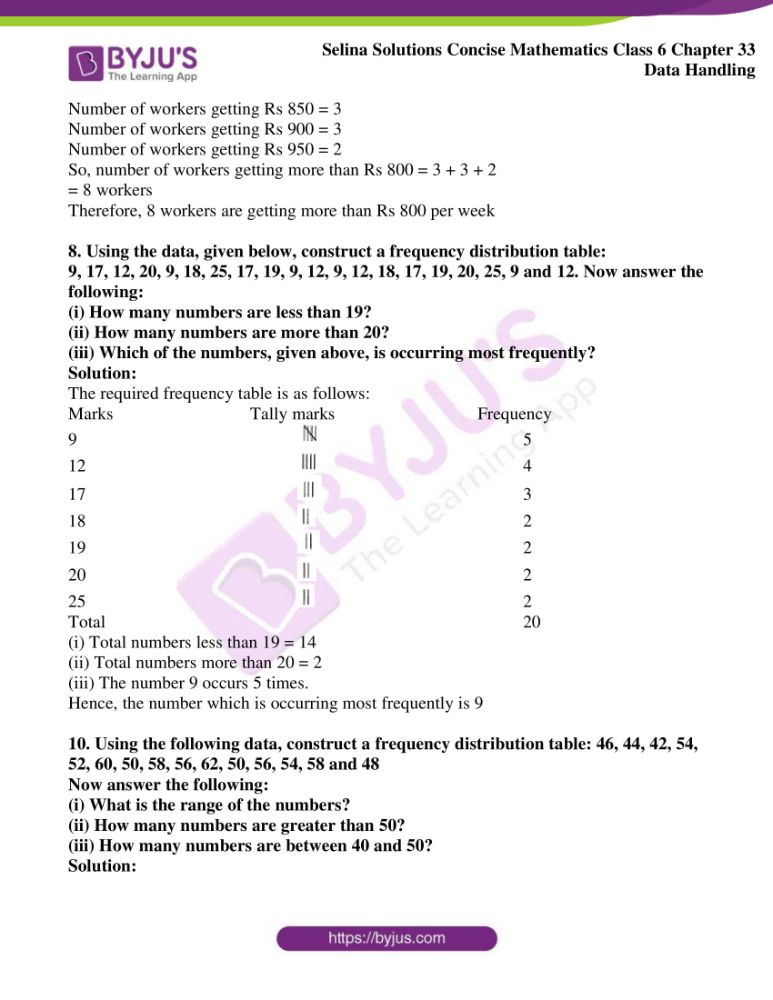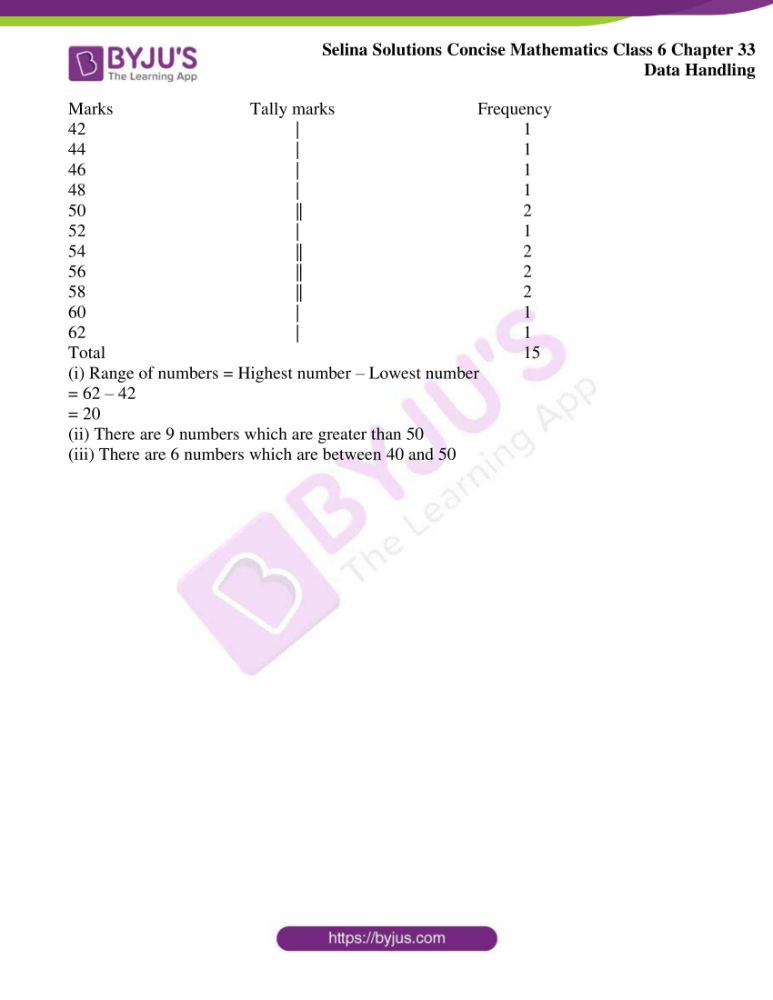### Access other exercises of Selina Solutions Concise Mathematics Class 6 Chapter 33 Data Handling

Exercise 33(B) Solutions

Exercise 33(C) Solutions

### Access Selina Solutions Concise Mathematics Class 6 Chapter 33 Data Handling Exercise 33(A)

Exercise 33(A)

1.Marks scored by 30 students of class VI are as given below:

38, 46, 33, 45, 63, 53, 40, 85, 52, 75, 60, 73, 62, 22, 69, 43, 45, 33, 47, 41, 29, 43, 37, 49, 83, 44, 55, 22, 35 and 45. State:

(i) the highest marks scored

(ii) the lowest marks scored

(iii) the range of marks

Solution:

(i) The highest marks scored is 85

(ii) The lowest marks scored is 22

(iii) Range of marks = Difference between highest and lowest marks

= 85 – 22

= 63

Therefore, the range of marks is 63

2. For the following raw data, form a discrete frequency distribution:

30, 32, 32, 28, 34, 34, 32, 30, 30, 32, 32, 34, 30, 32, 32. 28, 32, 30, 28, 30, 32, 32, 30, 28 and 30

Solution:

The required frequency table is shown below

Marks    Tally marks    Frequency

28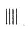4

30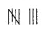8

32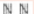10

34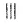3

Total                                    25

3. Define:

(i) data

(ii) frequency of an observation

Solution:

(i) Data: Information in the form of numerical figures is known as data

(ii) Frequency of an observation: The number of times a particular observation occurs is known as its frequency

4. Rearrange the following raw data in descending order:

5.3, 5.2, 5.1, 5.7, 5.6, 6.0, 5.5, 5.9, 5.8, 6.1, 5.5, 5.8, 5.7, 5.9 and 5.4. Then write the:

(i) highest value

(ii) lowest value

(iii) range of values

Solution:

The given numbers in descending order are as follows:

6.1, 6.0, 5.9, 5.9, 5.8, 5.8, 5.7, 5.7, 5.6, 5.5, 5.5, 5.4, 5.3, 5.2, 5.1

(i) Hence, the highest value is 6.1

(ii) Hence, the lowest value is 5.1

(iii) Range of values = Difference between highest value and lowest value

Hence,

Range of values = highest value – lowest value

= 6.1 – 5.1

= 1.0

5. Represent the following data in the form of a frequency distribution:

52, 56, 72, 68, 52, 68, 52, 68, 52, 60, 56, 72, 56, 60, 64, 56, 48, 48, 64 and 64

Solution:

The required frequency table for the given data is as follows:

Marks    Tally marks    Frequency

48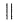2

52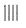4

56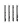4

60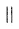2

64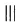3

68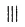3

72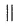2

Total                                     20

6. In a study of number of accidents per day, the observations for 30 days were obtained as follows:

6 3 5 6 4 3 2 5 4 2

4 0 5 3 6 1 5 5 2 6

2 1 2 2 0 5 4 6 1 6

Construct a suitable frequency distribution table.

Solution:

The required frequency table is shown below:

No. of accidents     Tally marks      Frequency

0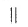2

1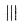3

2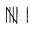6

3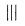3

4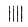4

5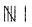6

6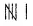6

Total                                                          30

7. The following data represents the weekly wages (in Rs) of 15 workers in a factory:

900, 850, 800, 850, 800, 750, 950, 900, 950, 800, 750, 900, 750, 800 and 850

Prepare a frequency distribution table. Now find,

(i) how many workers are getting less than Rs 850 per week?

(ii) how many workers are getting more than Rs 800 per week?

Solution:

The required frequency table is as follows:

Weekly wage (in Rs.)        Tally marks     Frequency

750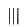3

800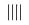4

850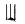3

900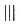3

950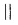2

Total no. of workers                                         15

(i) Number of workers getting less than Rs 850 per week are,

Number of workers getting Rs 750 = 3 workers

Number of workers getting Rs 800 = 4 workers

Hence, workers getting less than Rs 850 = 4 + 3

= 7 workers

Therefore, 7 workers are getting less than Rs 850 per week

(ii) Number of workers getting more than Rs 800 per week are,

Number of workers getting Rs 850 = 3

Number of workers getting Rs 900 = 3

Number of workers getting Rs 950 = 2

So, number of workers getting more than Rs 800 = 3 + 3 + 2

= 8 workers

Therefore, 8 workers are getting more than Rs 800 per week

8. Using the data, given below, construct a frequency distribution table:

9, 17, 12, 20, 9, 18, 25, 17, 19, 9, 12, 9, 12, 18, 17, 19, 20, 25, 9 and 12. Now answer the following:

(i) How many numbers are less than 19?

(ii) How many numbers are more than 20?

(iii) Which of the numbers, given above, is occurring most frequently?

Solution:

The required frequency table is as follows:

Marks        Tally marks         Frequency

9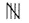5

12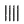4

17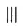3

18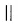2

19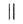2

20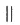2

25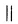2

Total                                             20

(i) Total numbers less than 19 = 14

(ii) Total numbers more than 20 = 2

(iii) The number 9 occurs 5 times.

Hence, the number which is occurring most frequently is 9

9. Using the following data, construct a frequency distribution table: 46, 44, 42, 54, 52, 60, 50, 58, 56, 62, 50, 56, 54, 58 and 48

(i) What is the range of the numbers?

(ii) How many numbers are greater than 50?

(iii) How many numbers are between 40 and 50?

Solution:

Marks          Tally marks         Frequency

42                         |                             1

44                         |                             1

46                         |                             1

48                         |                             1

50                        ||                            2

52                         |                             1

54                        ||                            2

56                        ||                            2

58                        ||                            2

60                         |                            1

62                         |                            1

Total                                                 15

(i) Range of numbers = Highest number – Lowest number

= 62 – 42

= 20

(ii) There are 9 numbers which are greater than 50

(iii) There are 6 numbers which are between 40 and 50